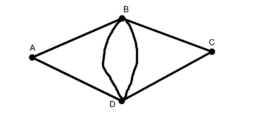# Searching for Eulerian and Hamiltonian Paths

Study each of the 6 graphs provided. (a) For each graph, find a Eulerian Path if one exists (i.e., a path for which each edge can be traversed exactly one time). (b) Next, find a Hamiltonian Path if one exists (i.e., a path that visits each vertex exactly once). (c) What do you notice about Graphs 4 and 5? (d) What do you notice about Graphs 3 and 6?
•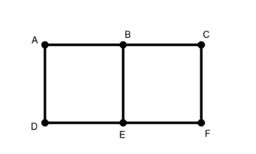### Graph 1

•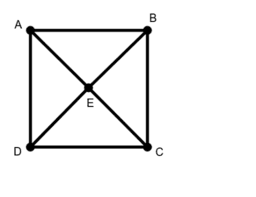### Graph 2

•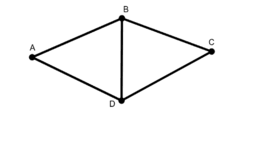### Graph 3

•### Graph 4

•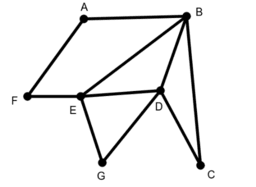### Graph 5

•